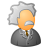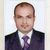# QlikView App Dev

Discussion Board for collaboration related to QlikView App Development.

Announcements
Join us at the Cloud Data and Analytics Tour! REGISTER TODAY
cancel
Showing results for
Search instead for
Did you mean:Not applicable

## Top ten in straight table with two dimensions

Hi,

I have a straight table with the dimensions "ConsigneeCity" and "Consignee", and as expression the waiting time at the consignee. I want my table to only show the ten highes values, but if I select top ten in the "dimension limits" tab, my table keeps going on blank and shows "Allocated memory exceeded". How do I still get the top ten?1 Solution

Accepted SolutionsPartner

Hello Frd ,

Use This Expression It Well Useful For U

To View It Top 10 City On 2 Dimensional Wise

if(aggr(Rank(Sum(waitingtime),10),ConsigneeCity)<=10,aggr(Sum(waitingtime),ConsigneeCity,Consignee))

6 RepliesMaster III

Hi Niek,

Please try this:

=aggr(if(Rank(sum(Your_Field))<=10,Your_Field),Your_Field) (For calculating Top 10)

Thanks,

ASMVP

Can you share your sample file please?Not applicable
Author

Do as Amit suggests in a calculated dimension then check Suppress When Value Is Null for the dimension in Settings for Selected Dimension.Partner

You can try this,

sum({<Value={"=rank(total aggr(sum(Value),Dim1,Dim2))<=\$(vL.SetTopValue)"}>}Value)

The expression below is something I use for a dynamic top X function in my tables/charts. But its for one dimension only,

(Where the user selects the wanted dimension from the data island - Field) (The dimension is a calculated dim using following, \$(=concat(Distinct Field)) )

sum({<\$(=concat(Distinct Field))={"=rank(total aggr(sum(Value),\$(=concat(Distinct Field))))<=\$(vL.SetTopValue)"}>}Value)MVP

For your dimension field use

Dimension1

Trim(ConsigneeCity)

Dimension2

Trim(Consignee)

Expression

Sum([Waiting time])

and in sort expression

Rank( sum( [Waiting time] ), 10 )

Hope this helpsPartner

Hello Frd ,

Use This Expression It Well Useful For U

To View It Top 10 City On 2 Dimensional Wise

if(aggr(Rank(Sum(waitingtime),10),ConsigneeCity)<=10,aggr(Sum(waitingtime),ConsigneeCity,Consignee))